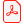# Konferensartikel

## Global Optimality Conditions for Discrete and Nonconvex Optimization; With Applications to Lagrangian Heuristics and Column Generation

Michael Patriksson
Chalmers University of Technology, Sweden

Ladda ner artikelhttp://www.ep.liu.se/ecp_article/index.en.aspx?issue=014;article=021

Linköping Electronic Conference Proceedings 14:21, s.

Visa mer +

ISBN:

ISSN: 1650-3686 (tryckt), 1650-3740 (online)

### Abstract

The well-known and established global optimality conditions based on the Lagrangian formulation of an optimization problem are consistent if and only if the duality gap is zero. We develop a set of global optimality conditions that are structurally similar but are consistent for any size of the duality gap. This system characterizes a primal-dual optimal solution by means of primal and dual feasibility; primal Lagrangian epsilonoptimality; and; in the presence of inequality constraints; delta-complementarity; that is; a relaxed complementarity condition. The total size epsilon + delta of those two perturbations equals the size of the duality gap at an optimal solution. The characterization is further equivalent to a near-saddle point condition which generalizes the classic saddle point characterization of a primal-dual optimal solution in convex programming. The system developed can be used to explain; to a large degree;when and why Lagrangian heuristics for discrete optimization are successful in reaching near-optimal solutions. Further; experiments on a set covering problem illustrate how the new optimality conditions can be utilized as a foundation for the construction of Lagrangian heuristics. Finally; we outline possible uses of the optimality conditions in column generation algorithms and in the construction of core problems; and illustrate our findings on instances of the generalized assignment problem.

### Nyckelord

Inga nyckelord är tillgängliga

### Referenser

Inga referenser tillgängliga